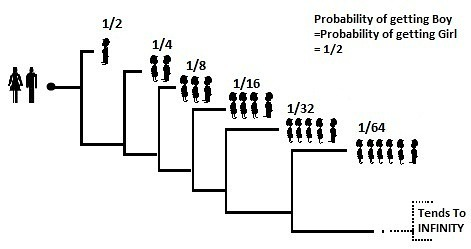# Ratio of Boys and Girls in a Country where people want only boys

1,399.0K Views

In a country,
All families wants a boy. They keep having babies till a boy is born.

What is the expected ratio of boys and girls in the country?Total Probability =ΣProbability of event  = 1/2 +1/4 +1/8 + …= 1

Now,
Expected no of Boys will be
= Σ Probability of event x Number of Boys in that event
=1/2 x1  + 1/4 x1  + 1/8 x 1  +  ….
= 1/2+1/4+1/8+1/16+…
= 1
Expected no of Girls will be
= Σ Probability of event x Number of Girls in that event
=1/2 x0  + 1/4 x1  + 1/8 x 2  + 1/16 x 3  ….         (See tree diagram)
= 1/4+ 2/8 + 3/16+ 4/32 …
=Sn
Sn          =     1/4+ 2/8 + 3/16+ 4/32  +…
1/2x Sn   =            1/8 + 2/16 + 3/32+ 4/64 +…   (Shifting all terms to right)
————————————————————-
Sn/2        =    1/4+ 1/8+ 1/16 + 1/32 +…              (Subtracting)

Sn/2 =  1/4 x 2
Sn = 1/4 x 2 x 2 =1

Expected no of Girls will be =1

Therefore
Ratio of no of Boys to Girls =
Expected no of Boys    = 1   = 1:1
Expected no of Girls       1

```Answer: The ratio is 1:1 (surprisingly)
Explanation: This is a simple question of probability...
Suppose we have C families. So we will eventually have C sons (after all, we keep giving birth until a son is born)
The probability of having a daughter is:
Number of girls = 0 * the probability of having 0 girls + 1 * the probability of having one girl + 2 * the probability of having 2 girls + ......
We will get: 0*(C*1/2) + 1*(C*1/2*1/2) + 2*(C*1/2*1/2*1/2) + …
That is: 0 + C/4 + 2*C/8 + 3*C/16 + …
It converges to C. So the ratio is 1:1```